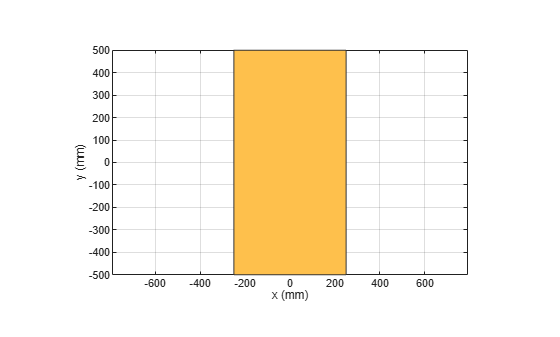# scale

Change size of shape by fixed factor

## Syntax

``scale(shape,factor)``
``c = scale(shape,factor)``

## Description

example

````scale(shape,factor)` scales the shape by a constant scaling factor and plots the resultant shape.```
````c = scale(shape,factor)` scales the shape by a constant scaling factor and returns a shape object.```

## Examples

collapse all

Create a rectangular shape.

```r = antenna.Rectangle; show(r) axis equal```Shrink the rectangle by 50%.

`scale(r,0.5);`## Input Arguments

collapse all

2-D and 3-D geometric shapes created using shape objects in Custom 2-D and 3-D Antenna catalog.

Example: `antenna.Rectangle`

Example: `shape.Polygon`

Example: `shape.Box`

Constant factor to change shape size, specified as a scalar.

Example: `2`

Data Types: `double`

## Output Arguments

collapse all

Resultant shape obtained after the scaling operation on the input shape, returned as a shape object.

Example: `antenna.Rectangle`

Example: `shape.Polygon`

Example: `shape.Box`

## Version History

Introduced in R2017a